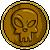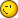# Getright Function

2 replies to this topic

### #1Colombia

Colombia

•• Members
•• 94 posts
• Gender:Male
• Location:Venezuela

• Calculators:
algebra FX 2.0 plus

Posted 06 June 2006 - 11:22 PM

How I can use the right part of any equation in Lua?

Ej.

y+4z+c=4+6j+9u

I just need "4+6j+9u"

And also, How I can store a equation???

I can`t do this

eqn=" y+4z+c=4+6j+9u"

I`ve two equal signs, How I can store this equation on a variable named eqn

### #2Orwell

Orwell

Casio Overlord

•• Members
•• 777 posts
• Gender:Male
• Location:Paris - France

• Calculators:
Casio AFX 1.02 / Casio ClassPad 300

Posted 06 June 2006 - 11:29 PM

How I can use the right part of any equation in Lua?

Ej.

y+4z+c=4+6j+9u

I just need "4+6j+9u"

```require "cas"
eq = cas("y+4z+c=4+6j+9u")   -- your equation, as a CAS object
print( cas.getRight(eq) )  -- prints the right part (it's a CAS object too)```

And also, How I can store a equation???

I can`t do this

eqn=" y+4z+c=4+6j+9u"

I`ve two equal signs, How I can store this equation on a variable named eqn

```require "cas"
eq = cas("y+4z+c=4+6j+9u")
cas(eq .. "=>eqn") -- '=>' is the assignment key```### #3Colombia

Colombia

•• Members
•• 94 posts
• Gender:Male
• Location:Venezuela

• Calculators:
algebra FX 2.0 plus